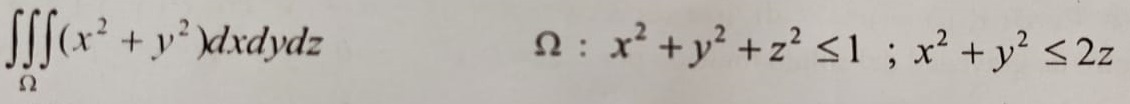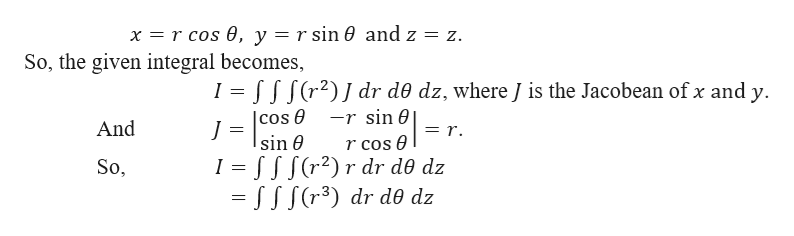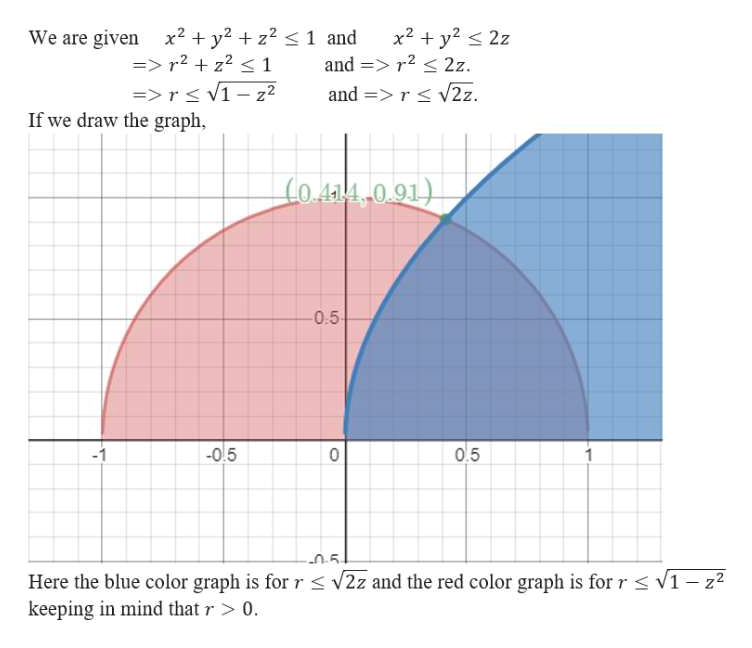Question
48 views

Use cylindrical or spherical coordinates to calculate the triple integralhelp_outlineImage TranscriptioncloseSx+ydxdydz 2 x +2+ <1 ; x2 +y 2z fullscreen
check_circle

Step 1

Here we will be using the cylindrical co-ordinate to find the following triple integral.

Step 2

By the cylindrical co-ordinate,help_outlineImage Transcriptioncloser cos 0, y =r sin 0 and z = z. x So, the given integral becomes, I fff(r2)J dr d0 dz, where ] is the Jacobean of x and y | cos e Isin 0 -r sin And =r. r cos I = fff(r2)r dr d0 dz = SS S(r3) dr d0 dz So, fullscreen
Step 3

Now, we need to find the limit...help_outlineImage TranscriptioncloseWe are given x2y2 z2 >r2 z2 < 1 =>rs v1 If we draw the graph, x2y2 2z and r2 2z. and =r v2z. 1 and z2 Co4a4 0.91) 0.5 0.5 -05 -1 5 Here the blue color graph is for r s v2z and the red color graph is for rs V1- z2 keeping in mind that r> 0 LO fullscreen

### Want to see the full answer?

See Solution

#### Want to see this answer and more?

Solutions are written by subject experts who are available 24/7. Questions are typically answered within 1 hour.*

See Solution
*Response times may vary by subject and question.
Tagged in

### Math